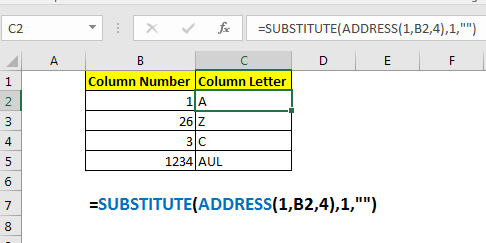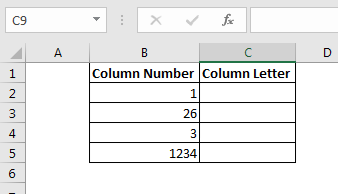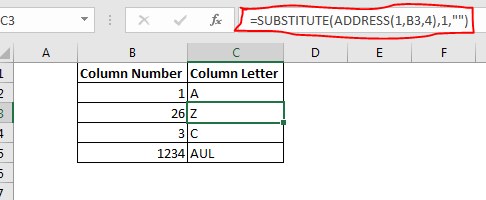# How to Convert Excel Column Number To Letter

Some times we get the need of converting given column number into the column letter (A, B, C,..), since the excel addressing function works with column letters.
So there’s no function that converts the excel column number to column letter directly. But we can combine SUBSTITUTE function with ADDRESS function to get the column letter using column index.Generic Formula

Column_number: the column number of which you want to get column letter.

Example: Covert excel number to column letter
Here we have some column numbers in B2:B5. We want to get corresponding column letter (A, B, C, etc) from that given number (1, 2, 3, etc.).Apply above generic formula here to get column letter from column number.

Copy down this formula. You have the column letters in C2:C5.How it works?
Well, this is a quite simple formula. The aim is to get first cell address of given column number. Then remove the row number to have only the column letter. We get the address of first cell of given column number using ADDRESS function.

ADDRESS(1,B2,4): Here B2 contains 1. This make translates to ADDRESS(1,1,4). The address FUNCTION returns the address at coordinate 1st row, 1st Column in relative format (4). This give us A1.

Now SUBSTITUTE function has SUBSTITUTE(A1,1,””). It replaces 1 with nothing (“”) from A1. This gives us A.

So yeah guys this how you can convert number to letter of column in excel. In our next tutorial, we will learn how to convert column letter to number. Till than, if you have any doubts regarding this article or any other topic in advanced excel, let us know in the comments section below.

Popular Articles:

The VLOOKUP Function in Excel

COUNTIF in Excel 2016

How to Use SUMIF Function in Excel

Terms and Conditions of use

The applications/code on this site are distributed as is and without warranties or liability. In no event shall the owner of the copyrights, or the authors of the applications/code be liable for any loss of profit, any problems or any damage resulting from the use or evaluation of the applications/code.